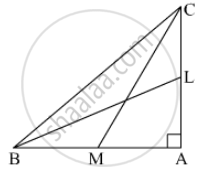# In the Given Figure, Bl and Cm Are Medians of a ∆Abc Right-angled at A. Prove that 4 (Bl2 + Cm2) = 5 Bc2. - Mathematics

Sum

In the given figure, BL and CM are medians of a ∆ABC right-angled at A. Prove that 4 (BL2 + CM2) = 5 BC2.#### Solution

To prove: 4(BL2 + CM2 ) = 5BC2

Proof: In ΔCAB,

Applying Pythagoras theorem,
AB2 + AC2 = BC2                                 .....(1)

In ΔABL,
AL2 + AB2 = BL2
⇒ ("AC"/2)^2 + "AB"^2 = "BL"^2

⇒ "AC"^2 + 4"AB"^2 = 4"BL"^2     .....(2)

In ΔCAM,
CA2 + MA2 = CM2
⇒ (("BA")/2)^2+ CA2 = CM2
⇒ BA2 + 4CA2 = 4CM2                     .....(3)

AC2 + 4AB2 + BA2 + 4CA2 = 4BL2 + 4CM2
⇒ 5AC2 + 5AB2 = 4(BL2 + CM2)
⇒ 5(AC2 + AB2) = 4(BL2 + CM2)
⇒ 5(BC2) = 4(BL2 + CM2)            ....( From 1 )
Hence Proved.

Concept: Right-angled Triangles and Pythagoras Property
Is there an error in this question or solution?